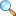# Chapter 05: Determinants

• Determinant of a square matrix
• Axiomatic definition of a determinant
• Determinant as sum of products of elements
• Determinant of the transpose
• An algorithm to evaluate Det A
• Determinants and inverse of matrices
• Exercise 5.1 |View online | Download PDF (1302KB)
• Exercise 5.2 |View online | Download PDF (396KB)
• bsc/notes_of_mathematical_method/ch05_determinants